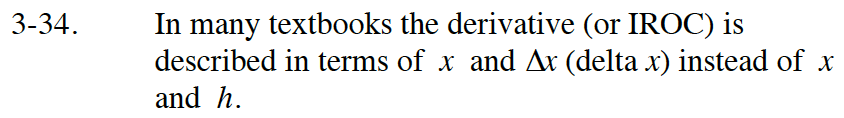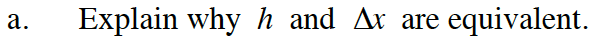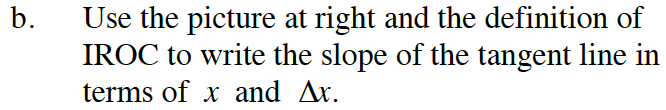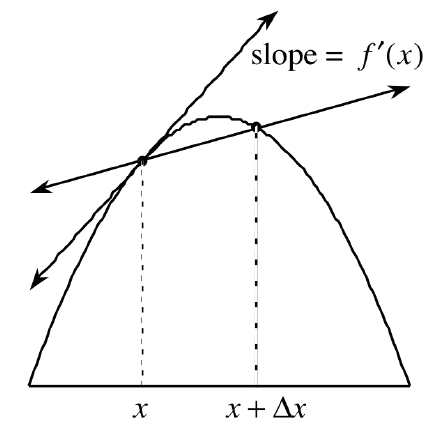### Home > CALC > Chapter Ch3 > Lesson 3.1.2 > Problem3-34

3-34.Δx can be translated as 'change in x.' h typically appears in the expression: (x + h) −x. How are these equivalent?'Definition of the Derivative' is the same thing as 'Instantaneous Rate of Change': IROC

$f'(x)=\lim_{h\rightarrow 0}\frac{f(x+h)-f(x)}{(x+h)-h}=\lim_{h\rightarrow 0}\frac{f(x+h)-f(x)}{h}...$